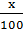### Sample Problem

Cody finds that his scatterplot of household income vs. SAT scores has a line of best fit with the equation y =+ 200. What does this line predict the SAT score for a student with a household income of \$195,000 to be?

#### Solution

The equation y =+ 200, when x=195,000

y =+ 200

y = 1950 + 200

y = 2150